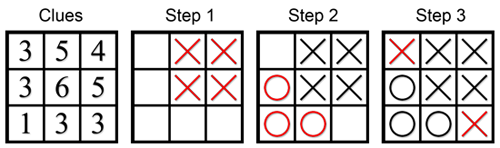## Directions:

Think-Tac-Toe is a puzzle where you are trying to discover the hidden locations of X’s and O’s in a grid by using number clues. The number in each square tells you the number of X’s in that square’s neighborhood. A square’s neighborhood is made up of the square itself and any squares with which it shares an edge or a corner.

## Example:Look at the #4 in the upper right hand corner of the Clues grid. It has a neighborhood of only four squares, so they must all be X’s (Step 1). Next, look at the #1 in the lower left hand corner. This square already has one X in its neighborhood, so there can’t be any more X’s. You can fill in O’s in the rest of its neighborhood (Step 2). Now, if you look at the #3 right above the #1 you can count the number of X’s already in its neighborhood. There are only two so far, so the square in the upper left must also have an X in it to make a total of 3 (Step 3). Similar logic will fill in the bottom right square, which completes this Think-Tac-Toe puzzle. There is only one correct answer. Try solving some more on the puzzles page!